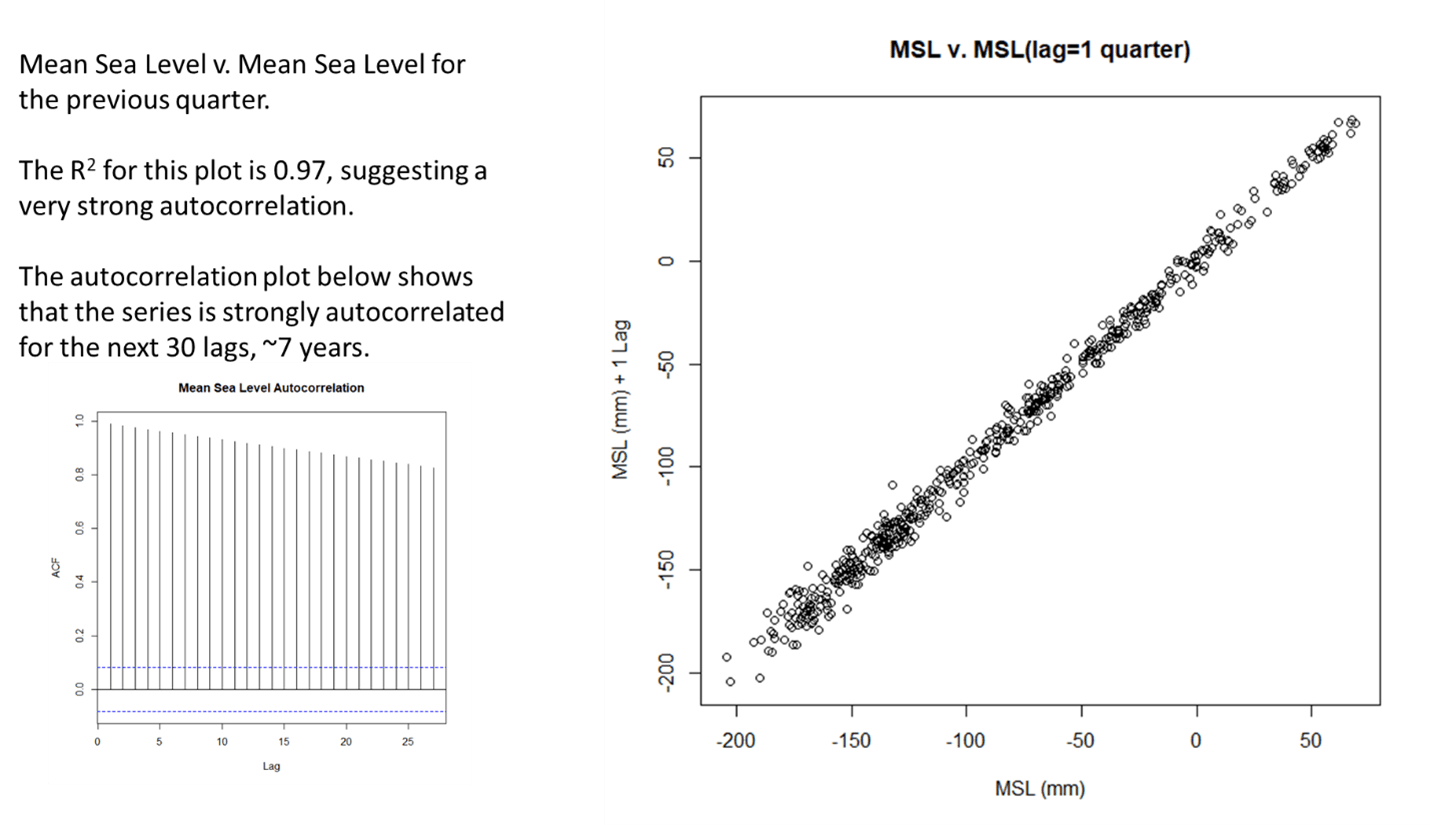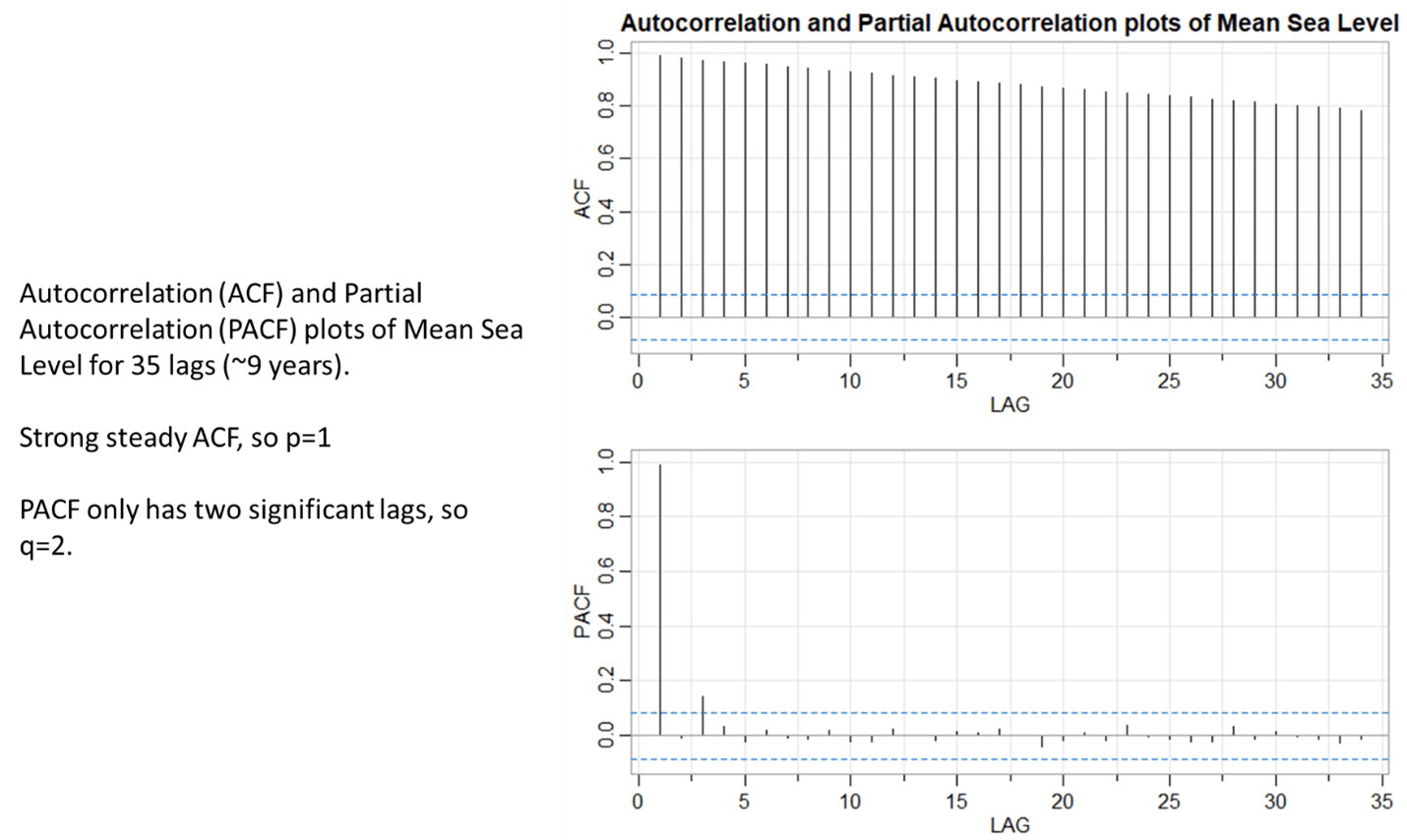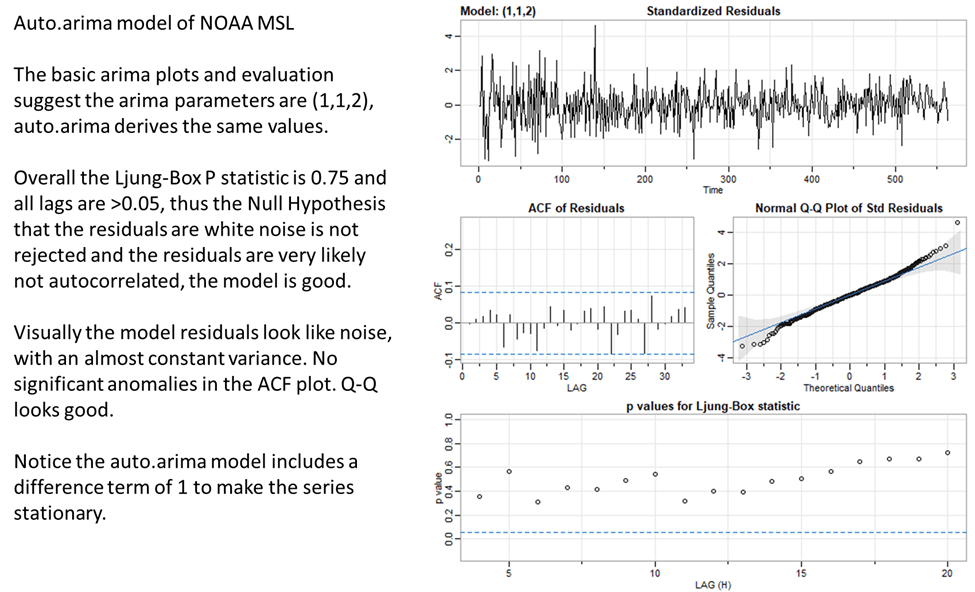# AR6 and Sea Level, Part 3, A Statistically Valid Forecast

By Andy May

In Part 1 of this series, we examined the data and analysis that was presented in AR6 to support their conclusion that sea level rise is accelerating. In Part 2 we looked at a serious examination of the observational record for sea level rise over the past 120 years and the modeled components of that rise. We concluded in Part 1 that the statistical evidence presented in AR6 for acceleration was crude and cherry-picked. In Part 2 we saw that the error in both the estimates of sea level rise and in estimating the components of that rise is very large. The error precluded determining acceleration with any confidence, but the data revealed an approximately 60-year oscillation of the rate of sea level rise that matches known natural ocean cycles.

Modern statistical tools allow us to forecast time series, like GMSL (global mean sea level) change, in a more valid and sophisticated way than simply comparing cherry-picked least squares fits as the IPCC does in AR6. Our forecast is based on pure statistics. It is done in the correct way, but not necessarily correct, statistics are like that. We will not know for sure until 2100. That said, let’s do it. If you have a certain kind of nerdy mind, you will enjoy this.

Figure 1 is a plot of the data we will use—the NOAA sea level dataset. Simply looking at it we can tell it is autocorrelated, which means that each quarter’s mean sea level estimate is highly dependent upon the previous quarter’s value. Autocorrelation is important to consider in least squares regression, especially when forecasting time series, but routinely ignored by the IPCC.Figure 1. NOAA mean sea level anomaly, 1900 to 2022. Each dot is one quarter (3 months).

Figure 2 plots each sea level estimate versus the previous estimate, this is called a plot of the first lag and the correlation of the two is a measure of autocorrelation. The R2 of the first lag is 0.97, so sea level is very autocorrelated. This is obvious but means that normal least squares linear fit statistics are invalid, the least squares statistics, such as R2, assume that the errors of regression are independent. Least squares, as used in AR6 to show acceleration, is inappropriate with a dataset like this. Most of any given value is heavily dependent upon the previous value. This means the mean-square-error (MSE) will be much too small, causing the error of the fit to be too small. As a result, any least squares line of the data in Figure 1 or any portion of that data is statistically useless, unless the autocorrelation is accounted for.Figure 2. Plot of GMSL versus the previous GMSL, the first lag. The values are highly correlated. The small autocorrelation plot shows that GMSL is highly autocorrelated for at least 7 years.

So how can we forecast GMSL in a statistically valid way? We clearly cannot use least squares and need to apply more advanced techniques. The first step is to remove the autocorrelation from the data, this is normally done by subtracting the previous GMSL value from the current one and progressing in this way throughout the data set. We have done this and show a plot of the result in Figure 3.Figure 3 A plot of the first difference of GMSL. The plot is random and fairly uniform left to right, suggesting that the autocorrelation is removed, and the data are stationary.

The first difference data from GMSL looks pretty good, very much like white noise. This is exactly what we want for valid statistical analysis and forecasting. We will be using an R function called “arima” to create our GMSL forecast, and this function requires three parameters to work, they are called p, d, and q. These parameters tell arima how to condition the input data and build a model that can project valid future values. The plot in Figure 3 shows us that “d” is one. That means taking one difference of adjoining values removes autocorrelation. We also need the data to be stationary, that is the statistical properties do not change with time (left to right). The original dataset (Figure 1) was clearly not stationary, and this is OK, we just do not want the way GMSL changes to be a function of time for this analysis. The R Augmented Dickey-Fuller Test (ADF) function confirms this, as the original dataset has an ADF p value of 0.79, meaning it is non-stationary. The arima p value is not the same as the statistical p test.

The differences plotted in Figure 3 have an ADF p value of 0.01, well below 0.05, the threshold needed to show stationarity. Data are stationary when the distribution over the period being studied is evenly distributed around the mean. That is the distribution, up and down, does not vary significantly with the time axis (x).

Next, we need to derive the arima p and q values. For this we need the ACF (autocorrelation) and PACF (partial autocorrelation) plots shown in Figure 4.Figure 4. ACF and PACF plots to determine p and q. The top plot shows that any value of one or over is possible for p since the series is very strongly autocorrelated at all lags. The lower plot shows that once the first level autocorrelation is removed only two significant autocorrelations remain (1 and 3) so q=2.

Analyzing the GMSL time series gives us an arima parameter set of (1,1,2) for (p,d,q). We can also run an R function called auto.arima to see what parameters it recommends. We find that it settles on (1,1,2) as well. This is good confirmation that our parameter selection is correct. Figure 5 plots the results.Figure 5. The results of an arima forecasting model. The top plot shows the residuals, next we see the ACF of the residuals and the Q-Q plot, both look good. The bottom plot gives the Ljung-Box statistics for various lags and they are all over 0.05, which means that the residuals are white noise, exactly what we want.

Figure 5 tells us that the model is successfully capturing the essence of the trends in mean sea level from 1880 through 2020. The model residuals show no trend and they are not autocorrelated. Figure 6 shows the arima forecast from the (1,1,2) model.Figure 6. The arima forecast of mean sea level to 2100. The confidence limits plotted are 95% limits. A histogram of the model residuals is shown to the lower left. The residuals are pleasingly normal.

Figure 7 is a plot of the forecast from Excel that is easier to read. The forecast we created predicts that GMSL will rise between 148 (6 inches) and 258 mm (10 inches) by 2100. Many researchers call this alarming, but humans have successfully adapted to much higher rates of sea level rise in the past as we can see in Figure 2 of Post 1, and they did so without the technology we have today. When we consider that the average open ocean daily tide range is 1,000 mm or three feet, eight inches of sea level rise over 100 years does not seem like much. In the 20th century sea level rose 5.5 inches, did anyone notice or care, aside from a few researchers?Figure 7. The forecast with more detail. The model predicts mean sea level in 2100 of 203 mm over the 1993-2008 average. The 95% confidence limits are 148 (6 inches) to 258 mm (10 inches) and marked with a curly brace. The range of predictions is not alarming, it is just over the 140 mm or 5.5 inches observed in the 20th century.

Conclusions

In the United States we would call the AR6 attempt to convince us that the rate of GMSL rise is accelerating, using adjoining cherry-picked least squares lines “high school,” meaning unsophisticated. Their method is problematic because GMSL is heavily autocorrelated and non-stationary, rendering their cherry-picked least squares fits and least squares statistics invalid.

Our fit, using the R function arima, is at least statistically valid. We specifically corrected for autocorrelation and forced the series to be stationary. We also addressed the minor partial autocorrelation that was left at one quarter and three quarters. The residuals of our model passed both the overall Ljung-Box test and multiple-lag Ljung-Box tests for white noise, meaning the arima model properly captured the 140-year trend in the NOAA sea level data.

Thus, while AR6 cherry-picked periods to support their conclusion that GMSL is accelerating, we reached the opposite conclusion using all the data in a statistically valid way. This does not mean that our forecast is correct, but it does mean that the AR6 speculation that sea level might rise 5 meters by 2150 is extremely unlikely and is best characterized as irresponsible speculation. Our analysis found no statistical evidence of acceleration and produced a linear extrapolation.

While warming of Earth’s surface is clearly the reason land-based glaciers are melting, which does contribute to rising sea level, AR6 provides no evidence the warming is caused by human activities. They use models to infer humans caused it, but unfortunately their models are also not statistically valid as shown in Part 2, here, and by McKitrick and Christy (McKitrick & Christy, 2018). We can all agree that humans probably have some impact on atmospheric warming, but we do not know how much is caused by humans and how much is natural, because we are emerging from the unusually cold Little Ice Age—the “preindustrial” period. Further, as we saw in Part 2, the 30-year rates of sea level rise reveal a distinctly natural-looking oscillation. Glacial ice and ice sheet melting is likely responsible for most of sea level rise, as AR6 states, but the human fraction of that warming might be quite small.

Thus, from a purely statistical point of view, the AR6 claims are childishly invalid. A proper analysis of the data leads to a forecast of roughly 20 cm (~8 inches) of sea level rise by 2100. In the year 2100, our descendants will know who was right.

The data and R code to create the figures in this chapter can be downloaded here. The R code and spreadsheet provide much more detail about the arima forecast, including references not supplied below.

# Works Cited

McKitrick, R., & Christy, J. (2018, July 6). A Test of the Tropical 200- to 300-hPa Warming Rate in Climate Models, Earth and Space Science. Earth and Space Science, 5(9), 529-536. Retrieved from https://agupubs.onlinelibrary.wiley.com/doi/full/10.1029/2018EA000401